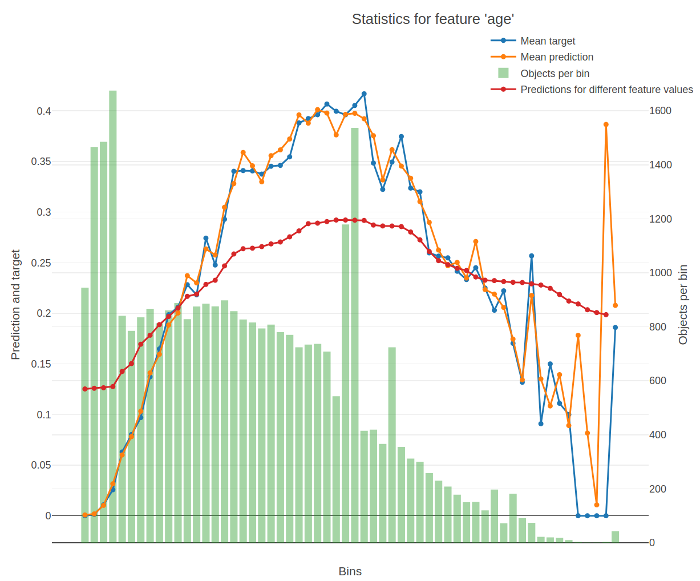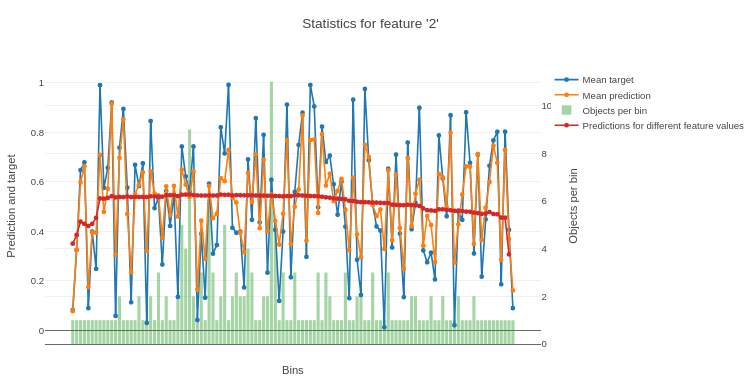# calc_feature_statistics

Calculate and plot a set of statistics for the chosen feature.

An example of plotted statistics:The X-axis of the resulting chart contains values of the feature divided into buckets. For numerical features, the splits between buckets represent conditions (feature < value) from the trees of the model. For categorical features, each bucket stands for a category.

The Y-axis of the resulting chart contains the following graphs:

• Average target (label) value in the bucket.

• Average prediction in the bucket.

• Number of objects in the bucket.

• Average predictions on varying values of the feature.

To calculate it, the value of the feature is successively changed to fall into every bucket for every input object. The value for a bucket on the graph is calculated as the average for all objects when their feature values are changed to fall into this bucket.

The return value of the function contains the data from these graps.

The following information is used for calculation:

• Trained model
• Dataset
• Label values
Restriction.
• Only models with one-hot encoded categorical features are supported. Set the one_hot_max_size parameter to a large value to ensure that one-hot encoding is applied to all categorical features in the model.
• Multiclassification modes are not supported.

## Method call format

``````calc_feature_statistics(data,
target=None,
feature=None,
prediction_type=None,
cat_feature_values=None,
plot=True,
max_cat_features_on_plot=10,
plot_file=None)``````

## Parameters

Parameter Possible types Description Default value
data
• numpy.array
• pandas.DataFrame

The data to calculate the statistics on.

Required parameter
target
• numpy.array
• pandas.Series

Label values for objects from the input data.

Required parameter
feature
• int
• string
• list of int, string or their combination

The feature index, name or a list of any their combination to calculate the statistics for.

Usage examples
Output information regarding a single feature indexed 0:
``feature=0``

Output information regarding two features, one of which is named `age` and the second is indexed 10:

``feature=["age", 10]``
Required parameter
prediction_type string

The prediction type to calculate the mean prediction.

Possible values:
• Probability
• Class
• RawFormulaVal
None (Probability for Logloss and RawFormulaVal, CrossEntropy for all other loss functions)
cat_feature_values
• list
• numpy.array
• pandas.Series

A list of categorical feature values to calculate the statistics on. Can be used if statistics are calculated for a categorical feature.

None (statistics are calculated for all values of categorical feature)
plot bool

Plot a Jupyter Notebook chart based on the calculated statistics.

True
max_cat_features_on_plot int

The maximum number of different values of a categorical feature to output on one chart.

If the selected categorical feature takes more different values than the value set in this parameter, several charts are plotted.

10

The number of threads to use for calculating statistics.

-1 (the number of threads is equal to the number of processor cores)
plot_file string The name of the output file to save the chart to. None (the file is not saved)
Parameter Possible types Description Default value
data
• numpy.array
• pandas.DataFrame

The data to calculate the statistics on.

Required parameter
target
• numpy.array
• pandas.Series

Label values for objects from the input data.

Required parameter
feature
• int
• string
• list of int, string or their combination

The feature index, name or a list of any their combination to calculate the statistics for.

Usage examples
Output information regarding a single feature indexed 0:
``feature=0``

Output information regarding two features, one of which is named `age` and the second is indexed 10:

``feature=["age", 10]``
Required parameter
prediction_type string

The prediction type to calculate the mean prediction.

Possible values:
• Probability
• Class
• RawFormulaVal
None (Probability for Logloss and RawFormulaVal, CrossEntropy for all other loss functions)
cat_feature_values
• list
• numpy.array
• pandas.Series

A list of categorical feature values to calculate the statistics on. Can be used if statistics are calculated for a categorical feature.

None (statistics are calculated for all values of categorical feature)
plot bool

Plot a Jupyter Notebook chart based on the calculated statistics.

True
max_cat_features_on_plot int

The maximum number of different values of a categorical feature to output on one chart.

If the selected categorical feature takes more different values than the value set in this parameter, several charts are plotted.

10

The number of threads to use for calculating statistics.

-1 (the number of threads is equal to the number of processor cores)
plot_file string The name of the output file to save the chart to. None (the file is not saved)

## Usage examples

``````from catboost import CatBoostRegressor
import numpy as np

train_data = np.random.rand(200, 10)
label_values = np.random.rand(200)

model = CatBoostRegressor()
model.fit(train_data, label_values)

res = model.calc_feature_statistics(train_data,
label_values,
feature=2,
plot=True)``````

The following is a chart plotted with Jupyter Notebook for the given example.